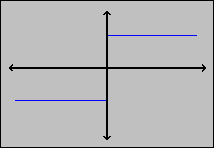#Interactive Real Analysis

Next | Previous | Glossary | Map

## 6.2. Continuous Functions

### Example 6.2.1:

Which of the two functions is intuitively continuous and which one is not ?
1. f(x) = 1 if x > 0 and f(x) = -1 if x < 0. Is this function continuous ?
2. f(x) = 5x - 6. Is this function continuous?
Since Descartes claims that a function is continuous if its graph can be drawn without lifting the pencil, we will look at the graph of each function:f(x) = -1 if x < 0 and 1 if x > 0: Since we have to lift the pencil to draw this graph, this function does not appear to be continuous.f(x) = 5x - 6: Since this graph, being a straight line, does not require us to lift the pencil, we would call this function continuous.
Next | Previous | Glossary | Map